# Weierstrass representation of a minimal surface

Letbe a Riemann surface. A harmonic conformal mappingthen defines a minimal surface in,(cf. also Harmonic function; Conformal mapping). Letbe local isothermal coordinates; thenSinceis harmonic,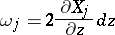is a holomorphic-form on. Hence any (branched) minimal surface incan be given bymeromorphic-forms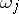satisfying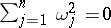, andcan be expressed as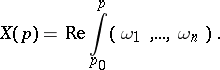(a1)

Such anis well defined onif and only if for any loopin,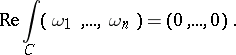(a2)

For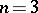, one gets a meromorphic functionand a meromorphic-form,On the other hand, given a meromorphic functionand a meromorphic-formon, define(a3)

then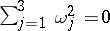. Thus, (a3) together with (a1) defines a minimal surface inand is called the Weierstrass representation of the minimal surface via the Weierstrass data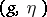.

The meromorphic functionhas the geometric meaning that it is the composite of the spherical mapping (or unit normal vector)and the stereographic projection from the north pole, where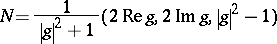andis also called the Gauss map of the minimal surface.

The first fundamental form and the Gaussian curvature of the surface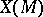can be expressed via,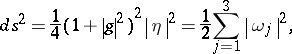Henceis a regular surface if and only ifon.

The second fundamental form ofcan be expressed as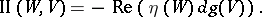Moreover,is an asymptotic direction if and only if, andis a principal curvature direction if and only if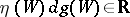.

The local Weierstrass representation was discovered in the 1860{}s by K. Enneper and K. Weierstrass. R. Osserman gave the general form on a Riemann surface in the 1960{}s, see [a1] for more details.